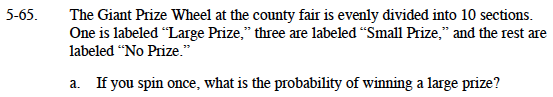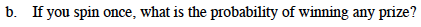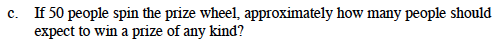### Home > MC1 > Chapter 5 > Lesson 5.2.3 > Problem5-65

5-65.Make a fraction using the given information.

The numerator will be the number of correct possible outcomes, and the denominator will be the number of total possible outcomes.Follow the steps in part (a).$\text{What number is }\frac{2}{5}\text{ of 50?}$

Use the eTool below to test the probability.
Click the link at right for the full version of the eTool: MC1 5-65 HW eTool